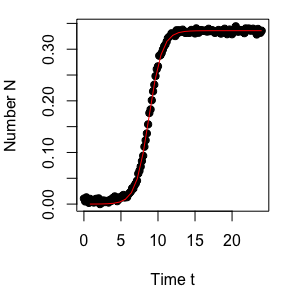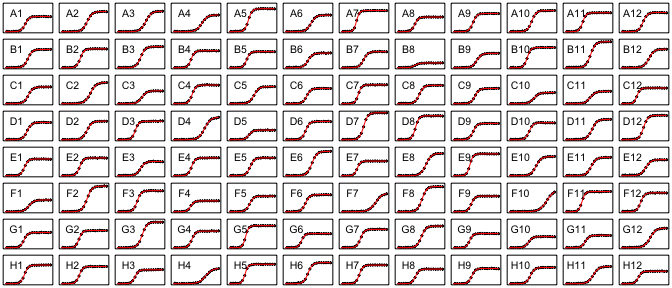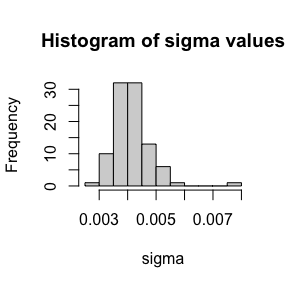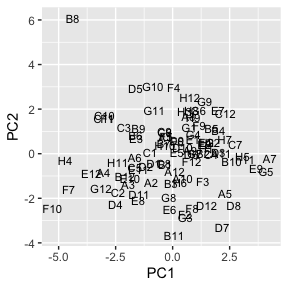# Using Growthcurver

## Introduction

Growthcurver calculates simple metrics to summarize growth curves.

Growth curves are commonly used in a variety of microbial experiments, including experimental evolution. The data are typically obtained by repeatedly measuring the cell density. Modern microbial growth curves can be conducted in a plate reader and may result in hundreds of absorbance measurements over the course of 24 hours.

In the Growthcurver package, we fit growth curve data to a standard form of the logistic equation common in ecology and evolution whose parameters (the growth rate, the initial population size, and the carrying capacity) provide meaningful population-level information with straight-forward biological interpretation. The logistic equation describes the population size $$N_t$$ at time $$t$$ using:

$\label{nt} N_t = \frac{K}{1 + \left( \frac{K-N_0}{N_0} \right) e^{-rt}} \$

Here, the population size at the beginning of the growth curve is given by $$N_0$$. The maximum possible population size in a particular environment, or the carrying capacity, is given by $$K$$. The intrinsic growth rate of the population, $$r$$, is the growth rate that would occur if there were no restrictions imposed on total population size. Growthcurver finds the best values of $$K$$, $$r$$, and $$N_0$$ for the growth curve data.

## Input data

We have provided simulated sample data in the simplest format for Growthcurver. The sample data that we’ve provided has one column for the time (in hours), and one column for each well in a 96-well plate. Each row contains the absorbance reading for each well at a given time, and the rows are sorted by time.

Preparing your data in this format will allow you to easily adapt our sample simple code, as well as the more complicated pipeline code, to your data.

Below, we show the first few rows and columns of the sample data.

time A1 B1 C1 D1 E1 F1 G1
0.0000000 0.0534859 0.0450445 0.0524558 0.0559717 0.0555779 0.0482403 0.0480286
0.1666667 0.0480034 0.0438943 0.0513760 0.0535398 0.0498355 0.0545188 0.0508498
0.3333333 0.0558745 0.0499971 0.0471483 0.0490218 0.0547812 0.0508200 0.0461799
0.5000000 0.0513175 0.0521323 0.0481636 0.0455630 0.0461267 0.0552204 0.0459967
0.6666667 0.0451672 0.0471601 0.0474251 0.0515392 0.0528446 0.0529912 0.0462149
0.8333333 0.0529321 0.0493626 0.0512493 0.0487991 0.0466157 0.0467418 0.0506130
1.0000000 0.0486773 0.0511002 0.0485762 0.0525684 0.0450770 0.0504815 0.0551161
1.1666667 0.0494391 0.0493021 0.0488559 0.0451704 0.0496254 0.0512137 0.0464252
1.3333333 0.0458304 0.0530621 0.0472789 0.0417502 0.0471547 0.0530060 0.0462033
1.5000000 0.0518993 0.0519433 0.0471127 0.0474941 0.0505342 0.0550318 0.0539161

Your data will probably come from the plate reader as an Excel spreadsheet. Convert the data in your spreadsheet so that the wells and time are the column headers and the data are the rows as shown above (Excel’s Paste Special | Transpose checkbox is useful for this). Save your growth curve data file as a tab-separated txt or csv file, and then read that file into R.

# Replace the next line with the location and name of your input data file.
file_name <- "the/path/to/my/data/myfilename.txt"
d <- read.table(file_name, header = TRUE, sep = "\t", stringsAsFactors = FALSE)

If you plan to analyze whole plates at a time, then the names of the columns of your input data must adhere to the following requirements * The column containing the time must be named time
* If you are doing background correction using measurements from a blank well, that well must be named blank (see the Background correction section for more details) * The remaining columns must have a unique well name that will be eventually be identified as the sample name

In this example, time is reported in units of hours. This means that all metrics involving time for these data are reported by Growthcurver in hours (e.g., $$r$$ is in hours$$^{-1}$$). If your data are in a different time unit than you would like, the following code may help you adjust the data in your time column.

# Convert the "time" column from hours to minutes
d$time <- d$time * 60

# Convert the "time" column from minutes to seconds
d$time <- d$time * 60

# Convert the "time" column from seconds to hours
d$time <- d$time / 60 / 60

### Background correction

Growthcurver provides two methods for doing a background correction to remove the absorbance signal due to the media. The default method (called min) finds the minimum value from each well, and subtracts it from all timepoints (for that well only). This method works well if you do not expect your background readings to change over time.

You can also select a second method (called blank), which is useful when the background readings change over time. This may happen, for example, when the media precipitates over the course of the growth curve. In this case, you provide the background correction data in a column called blank, which contains a series of measurements in a single media-only well measured at the same timepoints that the experimental measurements were made.

Finally, you can do your own background correction to the data before you call Growthcurver. In this case, you specify that you do not need Growthcurver to do any background correction for you (called none). I have provided two examples of custom background correction in the example code for Customize growth curves for a plate.

## A simple first example

In this example, we will use Growthcurver to summarize a single growth curve in a single well.

# First, load the package.
library(growthcurver)

# Load the sample growth curve data provided in the Growthcurver package.
# The first column is the time in hours, and there is one column
# for each well in a 96-well plate.
d <- growthdata

# Now, we'll use Growthcurver to summarize the growth curve data using the
# simple background correction method (minimum value correction). This is the
# default method, so we don't need to specify it in the command.
# This returns an object of type "gcfit" that holds information about
# the best parameters, the model fit, and additional metrics summarizing
# the growth curve.
gc_fit <- SummarizeGrowth(d$time, d$A1)

# It is easy to get the most useful metrics from a gcfit object, just type:
gc_fit
## Fit data to K / (1 + ((K - N0) / N0) * exp(-r * t)):
##      K   N0  r
##   val:   0.336   0   1.119
##   Residual standard error: 0.004685978 on 142 degrees of freedom
##
## Other useful metrics:
##   DT 1 / DT  auc_l   auc_e
##   0.62   1.6e+00 5.11    5.15
# And it is easy to plot the raw data and the best fit logistic curve
plot(gc_fit)## Output metrics

The object returned from SummarizeGrowth contains the metrics, and you can view them easily (as you did above), or you can manipulate them with R commands (as we will do now).

# The gcfit object returned from SummarizeGrowth also contains further metrics
# summarizing the growth curve data.
gc_fit$vals # look at the structure of the gc_fit object str(gc_fit) You’ll see that there are three main parts of a gcfit object: the vals, the model, and the data. vals contains the summarized metrics for your growth curver, model contains the details of the model fit (for advanced users), and data contains the input data to SummarizeGrowth. For most purposes, vals is the most useful. Let’s see what else we can access in the vals. # To see all the available metrics str(gc_fit$vals)
## List of 16
##  $k : num 0.336 ##$ k_se : num 0.000552
##  $k_p : num 1.65e-244 ##$ n0   : num 1.82e-05
##  $n0_se: num 2.42e-06 ##$ n0_p : num 5.59e-12
##  $r : num 1.12 ##$ r_se : num 0.0151
##  $r_p : num 3.57e-115 ##$ sigma: num 0.00469
##  $df : num 142 ##$ t_mid: num 8.78
##  $t_gen: num 0.62 ##$ auc_l: num 5.11
##  $auc_e: num 5.15 ##$ note : chr ""
##  - attr(*, "class")= chr "gcvals"
# To access a single metric (for example the growth rate r)
gc_fit$vals$r
##  1.118657

The most useful values are k, n0, and r, which are the values of the parameters for the logistic equation that best fit the data. The fitting algorithm provides a measure of uncertainty for each, which is available (for n) in the n_p and n_se values, for example. The values sigma and df are both determined during the nonlinear regression fit. Df is the degrees of freedom and sigma is a measure of the goodnesss of fit of the parameters of the logistic equation for the data; it is the residual standard error from the nonlinear regression model. Smaller sigma values indicate a better fit of the logistic curve to the data than larger values.

t_mid is the time at which the population density reaches $$\frac{1}{2}K$$ (which occurs at the inflection point), t_gen is the fastest possible generation time (also called the doubling time), auc_l is the area under the logistic curve obtained by taking the integral of the logistic equation, and auc_e is the empirical area under the curve which is obtained by summing up the area under the experimental curve from the measurements in the input data. If you decide to use auc_l or auc_e, make sure that you specify the parameter t_trim so that these metrics are comparable across samples or plates that were grown for different lengths of time.

The note value provides additional information about problems with fitting the logistic curve to your data. No common problems were identified if it is empty.

## Get growth curves for a plate

One often measures growth curves in a plate reader for many wells at the same time. Following is some sample R code that uses Growthcurver to summarize the growth curve data for a whole plate.

# First, load the package and the sample dataset.
library(growthcurver)
d <- growthdata
# To analyze your data from Excel, you should read your data into the variable
# called d. To do so, replace the next line with the name and location of
file_name <- "the/path/to/my/data/myfilename.txt"
d <- read.table(file_name, header = TRUE, sep = "\t", stringsAsFactors = FALSE)

# Make sure that you have a column called "time" (and a column called "blank"
# if you are using "blanks" for your background correction). See the
# "Input Data" data section of the Vignette if you need help with this.
# Now, we'll use Growthcurver to summarize the growth curve data for the entire
# plate using the default background correction method ("min").
gc_out <- SummarizeGrowthByPlate(d)
# If you would like to use the "blank" background correction, then call
# Growthcurver as follows
gc_out <- SummarizeGrowthByPlate(d, bg_correct = "blank")

# If you would like to generate plots for all of the growth curves in your
# plate, then call Growthcurver as follows. You can change the name of
# the output file "gc_plots.pdf" to something that makes sense for you.
gc_out <- SummarizeGrowthByPlate(d, plot_fit = TRUE,
plot_file = "gc_plots.pdf")

# The summary information for each well is listed as a row in the output
# data frame called gc_out.

# We can look at the first few rows in the output using the head command.
head(gc_out)
sample k n0 r t_mid t_gen auc_l auc_e sigma note
A1 0.3358696 1.82e-05 1.1186573 8.779414 0.6196242 5.112115 5.151667 0.0046860
B1 0.4041318 1.54e-05 1.0223886 9.949513 0.6779684 5.678234 5.718521 0.0044383
C1 0.3706032 1.76e-05 0.9865605 10.090879 0.7025896 5.154747 5.191147 0.0044099
D1 0.3819837 1.95e-05 1.0257106 9.635499 0.6757727 5.486987 5.538353 0.0054090
E1 0.3700136 1.50e-05 1.1968446 8.447183 0.5791455 5.754740 5.780758 0.0038871
# Or, you can save the entire data table to a tab-separated file that can be
# imported into Excel.
output_file_name <- "the/path/to/my/data/myfilename.txt"
write.table(gc_out, file = output_file_name,
quote = FALSE, sep = "\t", row.names = FALSE)

See the section Output metrics for more details on interpreting parameters.

## Customize growth curves for a plate

Advanced users may want more control over background correction or plotting of the curves. You can use the following code to customize summarizing your growth curves. To adapt this code to your data, just ensure that your data are in the same format as the example data (discussed previously in the Input data section). Most importantly, this code assumes that the time information is stored in a column named time.

Here, we will first create a data frame in which to store the output data. We will then loop through the columns in the experimental growth curve data, call SummarizeGrowth for each, and store the metrics for each column in the output data frame.

# As in the simple example, load the package and the data.
library(growthcurver)
d <- growthdata

# Let's create an output data frame to store the results in.
# We'll create it so that it is the right size (it's faster this way!),
# but leave it empty.
num_analyses <- length(names(d)) - 1
d_gc <- data.frame(sample = character(num_analyses),
k = numeric(num_analyses),
n0  = numeric(num_analyses),
r = numeric(num_analyses),
t_mid = numeric(num_analyses),
t_gen = numeric(num_analyses),
auc_l = numeric(num_analyses),
auc_e = numeric(num_analyses),
sigma = numeric(num_analyses),
stringsAsFactors = FALSE)

# Truncate or trim the input data to observations occuring in the first 20 hours.
# Remember that the times in these sample data are reported in hours. To use
# minutes (or to trim at a different time), change the next line of code.
# For example, if you still would like to trim at 20 hours, but your time data
# are reported in minutes use: trim_at_time <- 20 * 60
trim_at_time <- 20

# Now, loop through all of the columns in the data frame. For each column,
# run Growthcurver, save the most useful metrics in the output data frame,
# and make a plot of all the growth curve data and their best fits.

# First, create a plot for each of the wells in the 96-well plate.
# Uncomment the next line to save the plots from your 96-well plate to a
# pdf file in the working directory.
# pdf("growthcurver.pdf", height = 8.5, width = 11)
par(mfcol = c(8,12))
par(mar = c(0.25,0.25,0.25,0.25))
y_lim_max <- max(d[,setdiff(names(d), "time")]) - min(d[,setdiff(names(d), "time")])

n <- 1    # keeps track of the current row in the output data frame
for (col_name in names(d)) {

# Don't process the column called "time".
# It contains time and not absorbance data.
if (col_name != "time") {

# Create a temporary data frame that contains just the time and current col
d_loop <- d[, c("time", col_name)]

# Do the background correction.
# Background correction option 1: subtract the minimum value in a column
#                                 from all measurements in that column
min_value <- min(d_loop[, col_name])
d_loop[, col_name] <- d_loop[, col_name] - min_value
# Background correction option 2: subtract the mean value of blank wells
#                                 over the course the experiment
#                                 (Replace B2, D8, G11 with the column
#                                  names of your media-only wells)
#d$blank <- apply(d[, c("B2", "D8", "G11")], 1, mean) #d$A1 <- d$A1 - d$blank

# Now, call Growthcurver to calculate the metrics using SummarizeGrowth
gc_fit <- SummarizeGrowth(data_t = d_loop[, "time"],
data_n = d_loop[, col_name],
t_trim = trim_at_time,
bg_correct = "none")

# Now, add the metrics from this column to the next row (n) in the
# output data frame, and increment the row counter (n)
d_gc$sample[n] <- col_name d_gc[n, 2:9] <- c(gc_fit$vals$k, gc_fit$vals$n0, gc_fit$vals$r, gc_fit$vals$t_mid, gc_fit$vals$t_gen, gc_fit$vals$auc_l, gc_fit$vals$auc_e, gc_fit$vals$sigma) n <- n + 1 # Finally, plot the raw data and the fitted curve # Here, I'll just print some of the data points to keep the file size smaller n_obs <- length(gc_fit$data$t) idx_to_plot <- 1:20 / 20 * n_obs plot(gc_fit$data$t[idx_to_plot], gc_fit$data$N[idx_to_plot], pch = 20, xlim = c(0, trim_at_time), ylim = c(0, y_lim_max), cex = 0.6, xaxt = "n", yaxt = "n") text(x = trim_at_time / 4, y = y_lim_max, labels = col_name, pos = 1) lines(gc_fit$data$t, predict(gc_fit$model), col = "red")
}
}# Uncomment the next line to save the plots from your 96-well plate to a file
# dev.off()

After running the above code, the summary metrics are available for each well (each column in the input data corresponds to a single well). Each column of absorbance data is summarized, and the summary is a row in the output data frame that we created.

# Look at the first few rows (samples) of data in the output data frame.
# (I'm only showing the first 4 rows of results, but you may want to see more.
#  You can either look at everything using the command "d_gc", or adjust the
#  number of rows displayed by changing the 4 to something else,
#  e.g., "d_gc[1:15,]").
d_gc[1:4, ]
##   sample       k    n0       r    t_mid   t_gen   auc_l   auc_e   sigma
## 1     A1 0.33586 2e-05 1.11875  8.77932 0.61957 3.76854 3.75229 0.00488
## 2     B1 0.40389 2e-05 1.02383  9.94781 0.67701 4.06000 4.03270 0.00470
## 3     C1 0.37075 2e-05 0.98563 10.09205 0.70325 3.67337 3.64760 0.00463
## 4     D1 0.38262 2e-05 1.02168  9.64025 0.67844 3.96389 3.95078 0.00572

## Quality control and best practices

Sometimes Growthcurver doesn’t find the best fit for your data. This can happen especially in the following cases:

• your population didn’t reach stationary phase during data collection,
• you don’t have enough measurements during the initial or final parts of the growth curve (lag phase or stationary phase),
• you didn’t do a background correction, or your background correction method doesn’t bring the minimum absorbance reading to near zero,
• you are trying to run Growthcurver on an empty well.

To see if Growthcurver has encountered any of these problems that can lead to a poor fit, we recommend doing these three quality control steps after you have run Growthcurver. Each step is covered in more detail below.

• Plot the curves and your data
• Check if Growthcurver returned any fit notes
• Identify outliers

### Plot the curves and your data

Firstly, we strongly recommend plotting all the curves and checking them manually. See the sections A simple first example and Get growth curves for a plate for code demonstrating how to easily plot your data and Growthcurver’s fit.

### Check for poor fit notes

Growthcurver returns a note if it finds a potential problem with the fit of the logistic curve to your data. This may happen when it cannot fit the logistic curve to your data, or if it finds evidence of a questionable fit. For example, Growthcurver returns a note when the carrying capacity $$K$$ is greater than the initial population size $$N_0$$, or when the inflection point t_mid is found to be negative (both things should not happen in a well-behaved growth curve!)

You can examine the note after fitting the growthcurves for an individual sample, or for an entire plate.

# Check if Growthcurver provided any notes in a plate of growthcurves returned
# from SummarizeGrowthByPlate
gc_out %>% filter(note != "")

# Check if Growthcurver provided any notes in a single growthcurve returned
# from SummarizeGrowth
gc_fit$vals$note

We simulated this dataset, so it doesn’t have any data noisy enough to cause problems with Growthcurver. Therefore, there are no notes returned for these samples.

### Identify outliers

You should look for outliers that have unusually large sigma values. Each sigma value is the residual sum of squares from the fit of the logistic curve to the data, so larger values mean poorer fits. To simplify looking at the sigma values, I use the package dplyr for data wrangling and exploration.

# Load dplyr and the sample output data
library(dplyr)
gc_out <- as_data_frame(gc_out)
## Warning: as_data_frame() is deprecated as of tibble 2.0.0.
## Please use as_tibble() instead.
## The signature and semantics have changed, see ?as_tibble.
## This warning is displayed once every 8 hours.
## Call lifecycle::last_warnings() to see where this warning was generated.
# Plot a histogram of the sigma values in order to check for outliers
hist(gc_out$sigma, main = "Histogram of sigma values", xlab = "sigma")# Show the top 5 samples with the largest sigma value # (with the worst model fit to the growth curve data) gc_out %>% top_n(5, sigma) %>% arrange(desc(sigma)) ## # A tibble: 5 x 10 ## sample k n0 r t_mid t_gen auc_l auc_e sigma note ## <chr> <dbl> <dbl> <dbl> <dbl> <dbl> <dbl> <dbl> <dbl> <chr> ## 1 E12 0.355 0.00003 0.863 10.7 0.803 4.71 4.79 0.00751 "" ## 2 B11 0.575 0.00002 0.941 11.1 0.736 7.40 7.46 0.00574 "" ## 3 D8 0.528 0.00002 1.28 8.02 0.543 8.44 8.49 0.0055 "" ## 4 D1 0.382 0.00002 1.03 9.64 0.676 5.49 5.54 0.00541 "" ## 5 G12 0.434 0.00002 0.779 12.6 0.889 4.93 4.99 0.00536 "" We simulated this dataset, so it is not very noisy. Therefore, it is not surprising that there aren’t any extreme sigma outliers in this case. One additional method for identifying outlier parameters is to conduct a principal components analysis on the samples, which projects high dimensional data on lower dimensional space. Plotting the samples on the first two principal components (PC1 and PC2) can identify natural clusters within the growth curve data as well as outliers. # Load dplyr, ggplot2, and the sample data library(dplyr) library(ggplot2) pca_gc_out <- as_data_frame(gc_out) # Prepare the gc_out data for the PCA rownames(pca_gc_out) <- pca_gc_out$sample
## Warning: Setting row names on a tibble is deprecated.
# Do the PCA
pca.res <- prcomp(pca_gc_out %>% select(k:sigma), center=TRUE, scale=TRUE)

# Plot the results
as_data_frame(list(PC1=pca.res$x[,1], PC2=pca.res$x[,2],
samples = pca_gc_out\$sample)) %>%
ggplot(aes(x=PC1,y=PC2, label=samples)) +
geom_text(size = 3)Most of the samples in this plot cluster together, except B8 which should be examined more closely to determine if it had a poor fit.